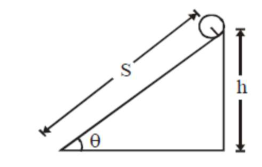# The following bodies,Question:

The following bodies,

of same mass ' $m$ ' and radius ' $R$ ' are allowed to roll down without slipping simultaneously from the top of the inclined plane. The body which will reach first at the bottom of the inclined plane is

[Mark the body as per their respective numbering given in the question]1. a ring

2. a disc

3. a solid cylinder

4. a solid sphere.

Correct Option: , 4

Solution:

(4)

$\mathrm{Mg} \sin \theta \mathrm{R}=\left(\mathrm{mk}^{2}+\mathrm{mR}^{2}\right)^{\alpha}$

$\alpha=\frac{R g \sin \theta}{k^{2}+R^{2}} \Rightarrow a=\frac{g \sin \theta}{1+\frac{k^{2}}{R^{2}}}$

$\mathrm{t}=\sqrt{\frac{2 \mathrm{~s}}{\mathrm{a}}}=\sqrt{\frac{2 \mathrm{~s}}{\mathrm{~g} \sin \theta}\left(1+\frac{\mathrm{k}^{2}}{\mathrm{R}^{2}}\right)}$

for least time, $\mathrm{k}$ should be least $\backslash$ we know $\mathrm{k}$

is least for solid sphere.